# Module 2: BASIC PROGRAMMING FUNDAMENTALS

## Learning Objectives

1. account for the programming concept of a data type,

2. convert between data types,

3. check the data type of any variable in MATLAB.

## What is a data type?

In computer programming, a data type is classification (or type) of the data you use to program. That is, MATLAB categorizes each variable or any piece of data into different groups. For example, MATLAB will treat a numeric integer (1, 5, 99, etc.) differently than an alphabetical character (“a”, “U”, etc.). Recall from Lesson 2.4 (Inputs and Outputs), we had to include in the fprintf() function what data type we were giving it (e.g., %s for string).

MATLAB refers to the different data types as fundamental MATLAB classes: MATLAB uses “data type” and “class” interchangeably. For someone familiar with object-oriented programming (C, C#, Java, etc.), this may be a little confusing. However, for the scope of this lesson, do not overthink this concept.

## What are the MATLAB data types?

While the range of data types that a programming language supports varies with the language, MATLAB solely works with six fundamental data types. Fundamental MATLAB data types are:

• Numeric - 1, 2, -54, 4.56

• Logical - True, False

• Character - ‘g’, ‘M’, ‘.’, ’ ’

• Cell - Can contain any data type

• Table - Data stored in tabular format

• Struct - Data related to groups using data containers called fields

While there are multiple data types available to MATLAB users, this course will focus on the most common: numeric, logical, and character/strings.

## Why are data types important?

The types of data (and therefore the “data types”) you will use in MATLAB will vary depending on their function and application. Some MATLAB functions only accept a specific data type, while other functions accept multiple forms of data. For example, MATLAB function fprintf() requires a character data type input (e.g., fprintf('hey there!')), while the mean() function requires numeric data types (e.g., mean(1.0, 2.5, 2.0, 3.0, 6.6)).

## How do I check the data type of a variable?

Let’s say you want to know the data type of a variable. For example, you want to probe the variable myVariable and find out its data type. This is achieved using the class() MATLAB function. Note that in the following example, the output is double, which is a sub-data type of the numeric class (data type).

### Example 1

Store the number 5.678 in a variable, and check the data type (class) of the variable.

Solution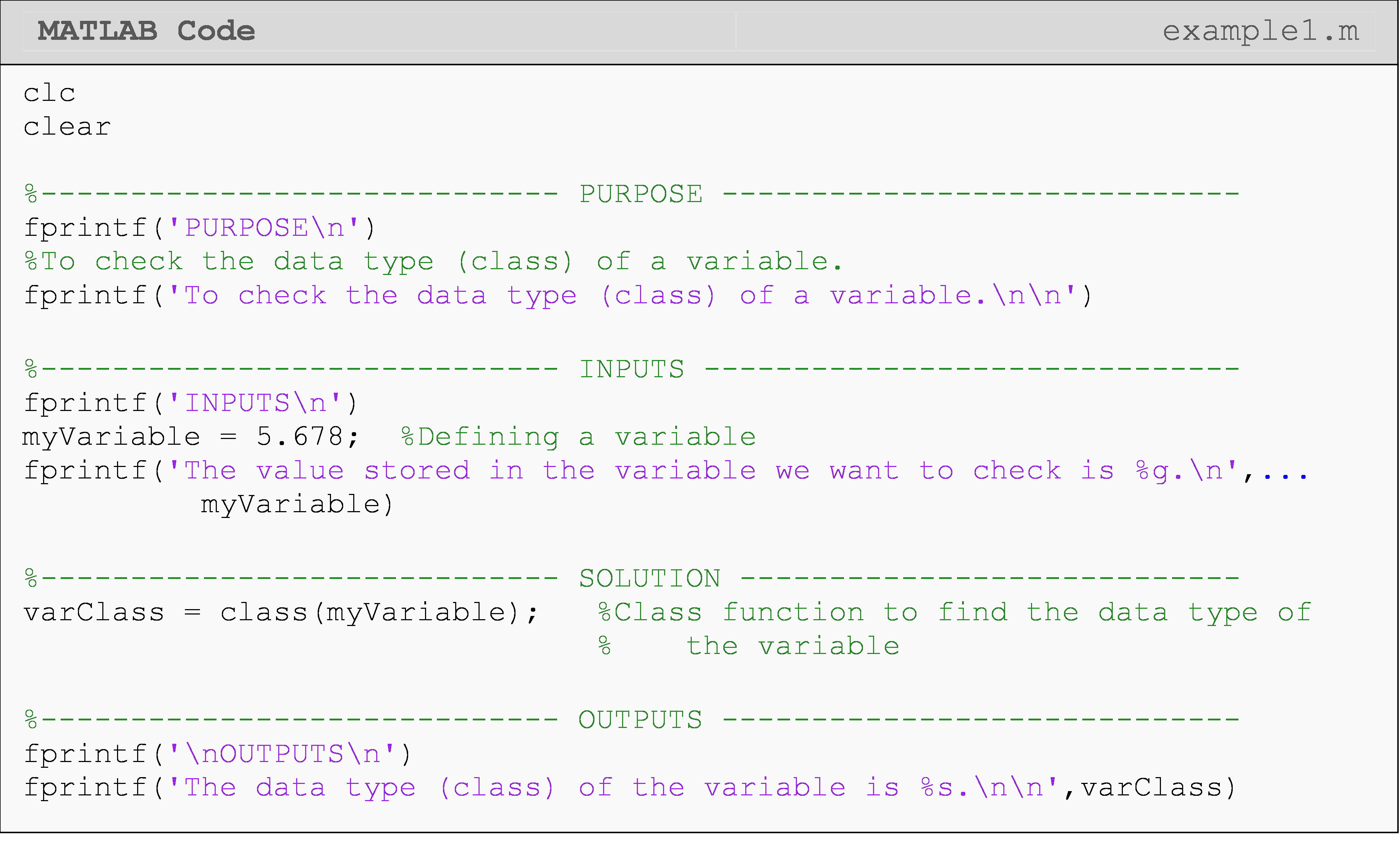As a side note, notice that there are three consecutive dots used in Example 1 so that the fprintf() function can be written on two lines. This will work in many other cases, which you will see in future examples. One important rule for using this is that it cannot be used in the middle of a string or a number.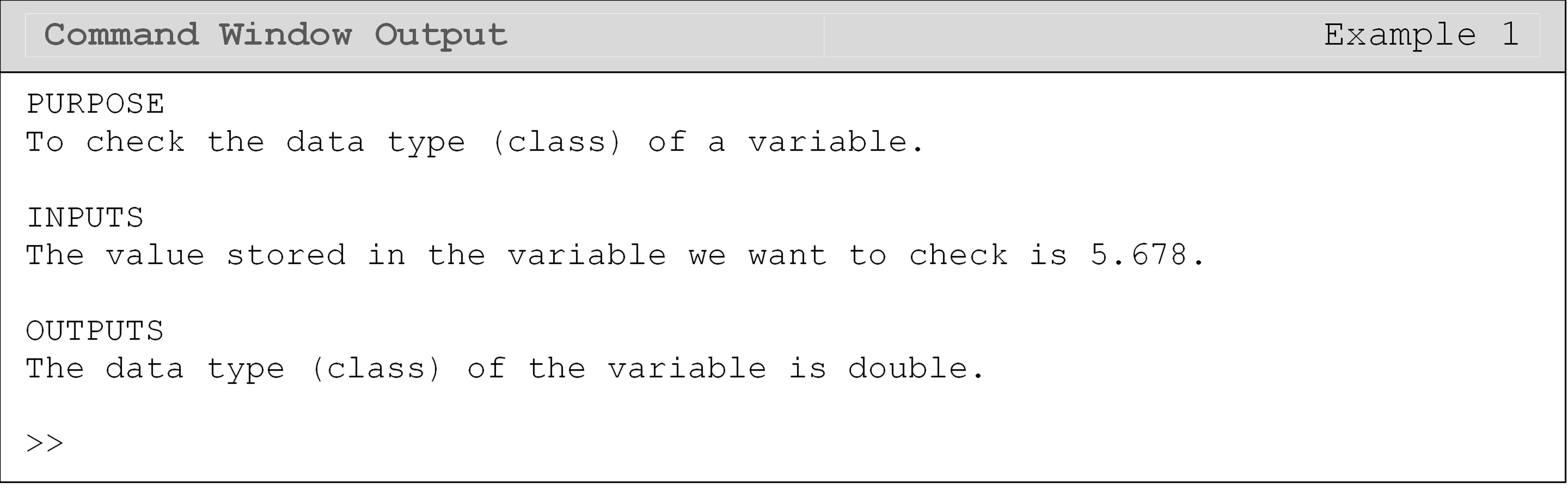You can ask MATLAB if the particular variable is a specific data type, where MATLAB will respond with either yes, true (1), or no, false (0). This is achieved using a variety of MATLAB data type identification functions.

One of these functions, ischar(), is used if you would like to see if a particular variable is a character data type. In the following example, a numeric double input of 5.678 into the ischar() function returns false, which means that 5.678 is not a character. On the other hand, notice that isnumeric() will return a true.

### Example 2

Conditionally check whether a variable is the char data type or a numeric data type.

Solution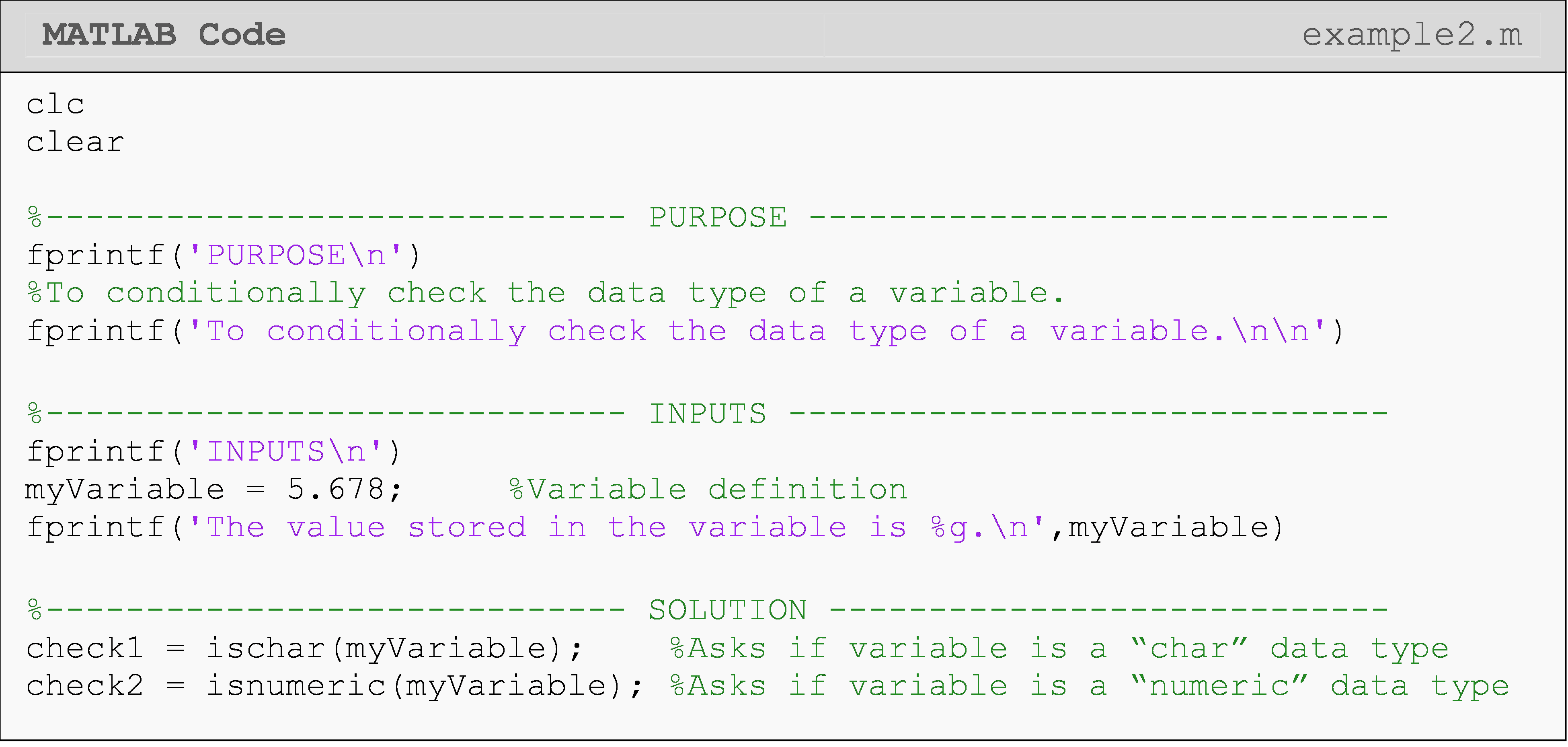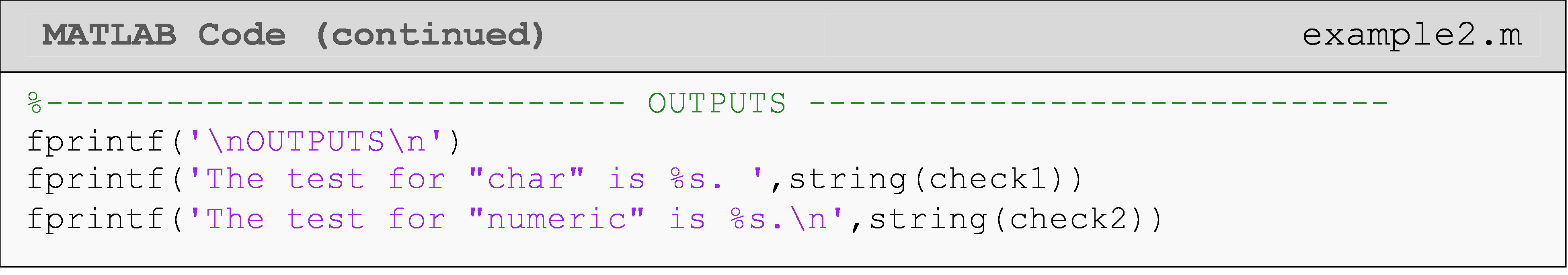The use of string() in the last two lines of the code is to convert the logical value (0 or 1) to “false” or “true”, respectively, for readability. You can see the output of this below. We will cover logical data types in Lesson 5.1; so, you do not need to worry about this conversion right now.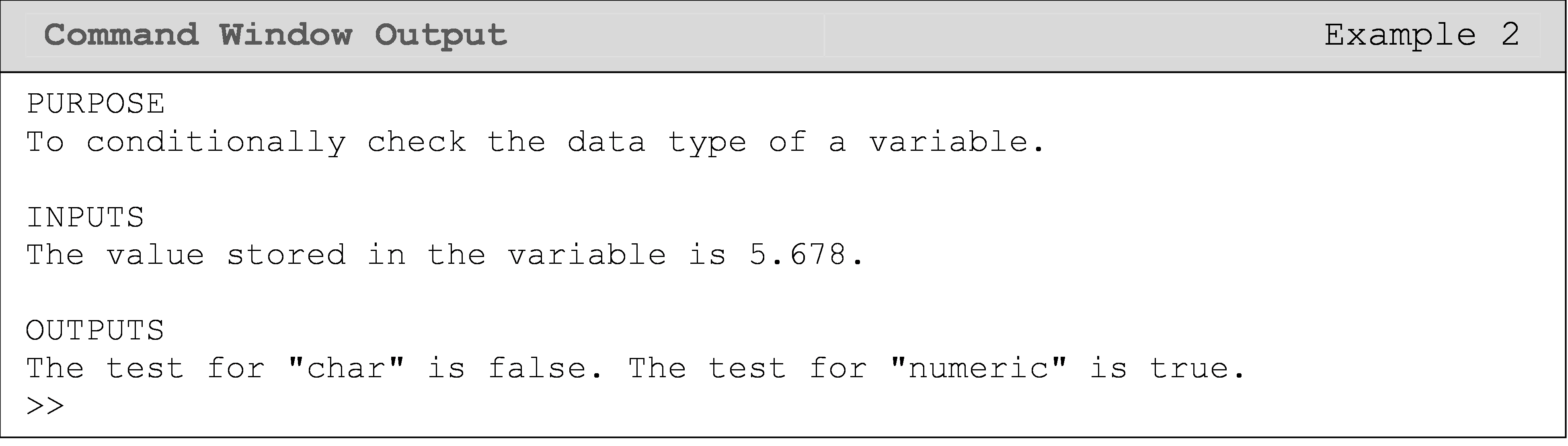## Can I convert between data types?

MATLAB provides functions to convert between most data types. Many of these have straightforward names like num2str(), which converts a number to a string, or character array. Others are simply the name of the MATLAB class like char() or double(). We will cover more of these functions as appropriate in the following lessons. You can review MATLAB documentation for a full list of data type conversion functions.

### Example 3

Convert the number 5.678 into a string.

Solution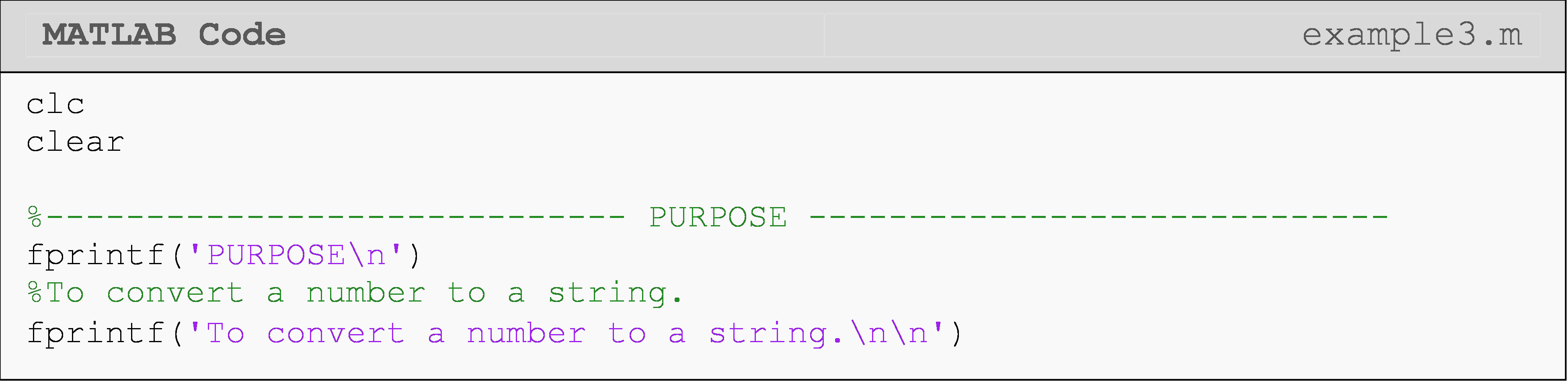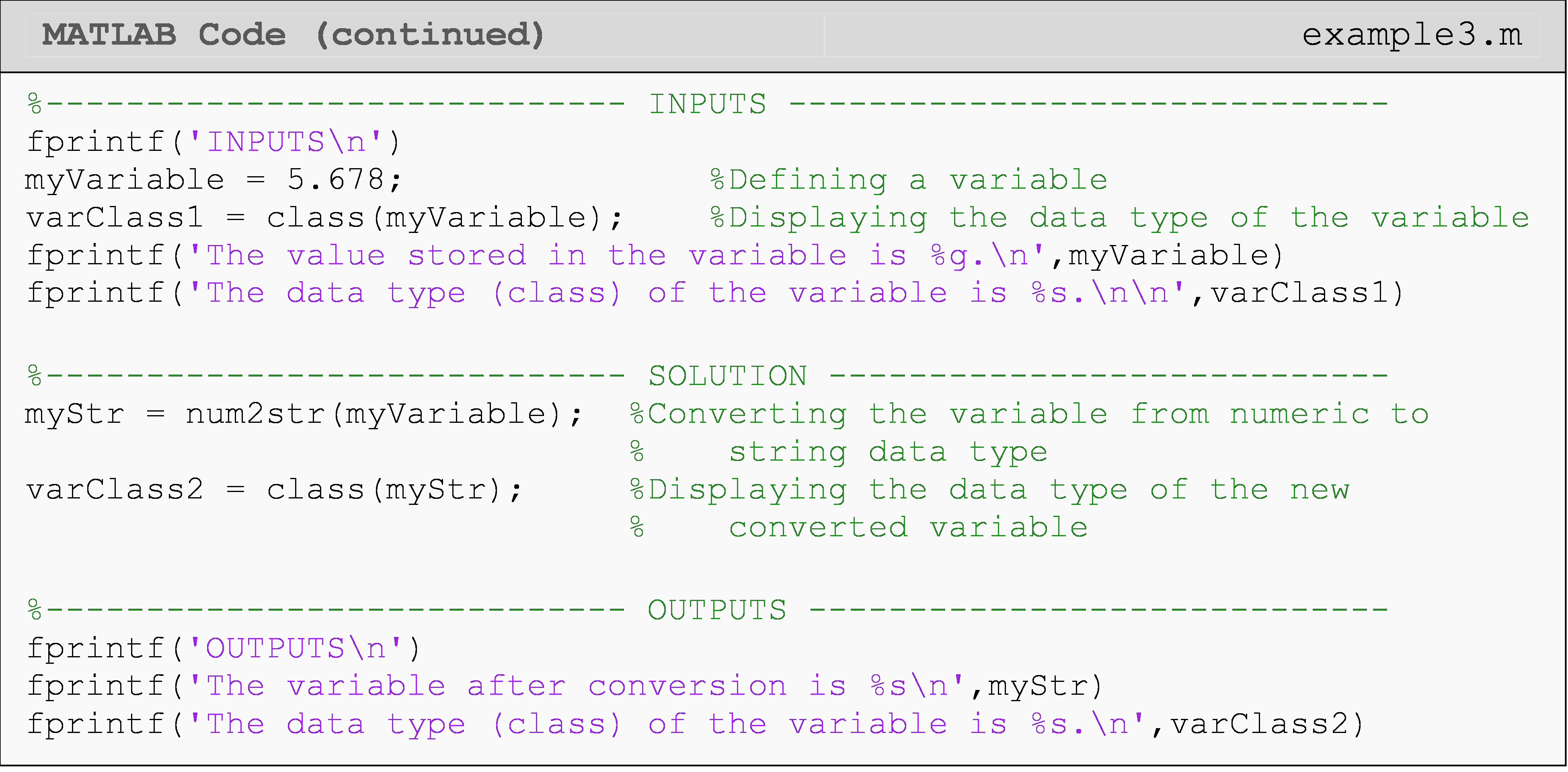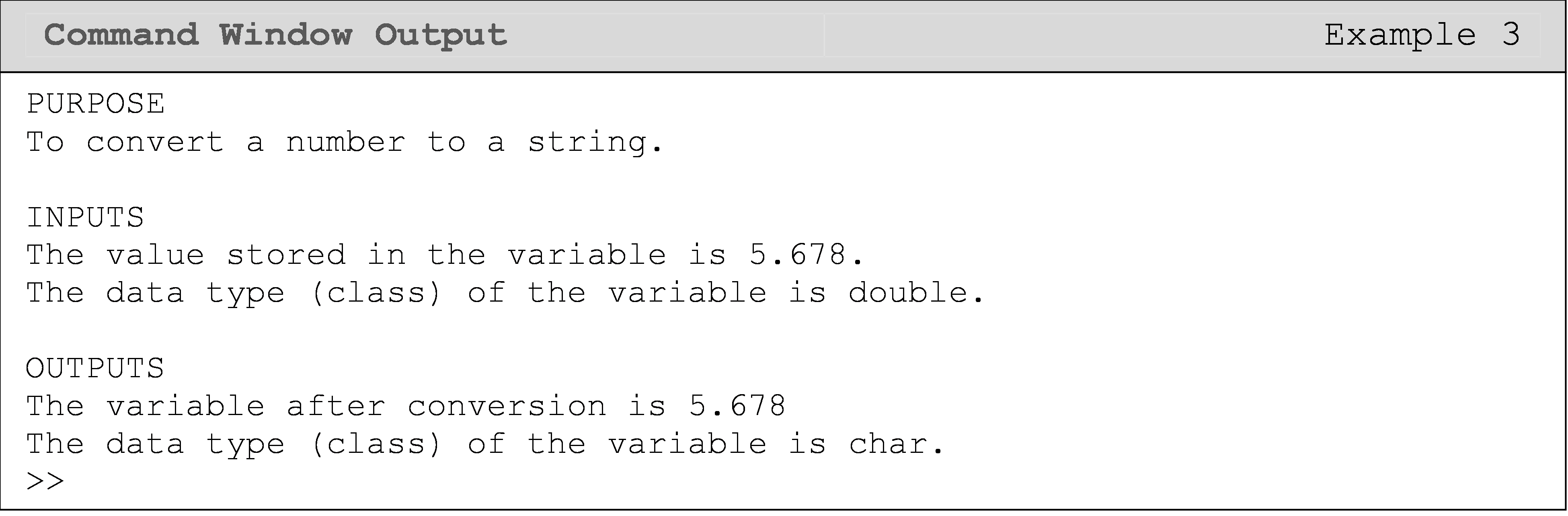Another common example of where data types become important is that you cannot directly join a string and a number to form a new string. To concatenate a number with other strings, you must first use the num2str() function on the number. The num2str() function converts a number to a string, thus making the number capable of joining with other strings. Conversely, to convert a string to a number use str2num() function. Though it should be noted that this will only work if the characters are numbers. The difference between numbers and characters that are numbers was covered in more detail in Lesson 2.2.

### Example 4

Input the following two strings and expression, str1, str2, and n, respectively into a new m-file.

str1 = 'Strings can tie'

str2 = 'or more knots'

n = 3

1. Convert the variable, n to a string and concatenate these three strings into a single sentence, “String can tie 3 or more knots.”, and output it to the Command Window using the fprintf() function.

2. Convert the variable, n back to a number and output the sentence “You are making at least 3 knots.” to the Command Window using the fprintf() function.

Solution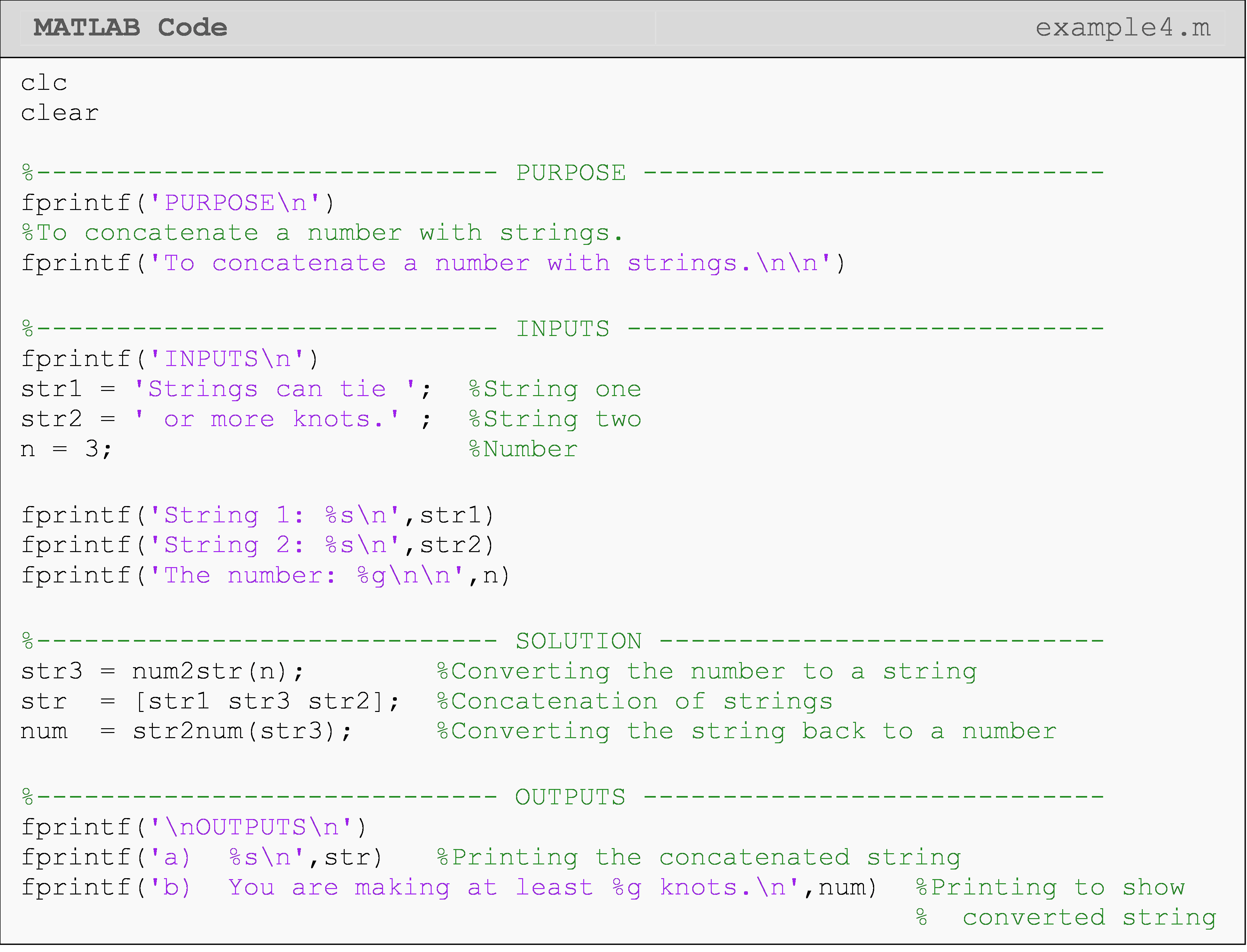You can see that the m-file in Example 4 uses three pieces to make a full sentence, where one of the pieces is defined as a number, n, and requires the use of the num2str() function to be concatenated with the two other strings. It must be converted to a string because all three pieces (variables) must be strings, or more precisely, they must all be of the char data type. The variable str3, which stores the character 3, is then converted “back” to a number data type, as a demonstration. This is accomplished using the str2num() function. The variable str3 is then a number and can be used with the fprintf() function and the %g format.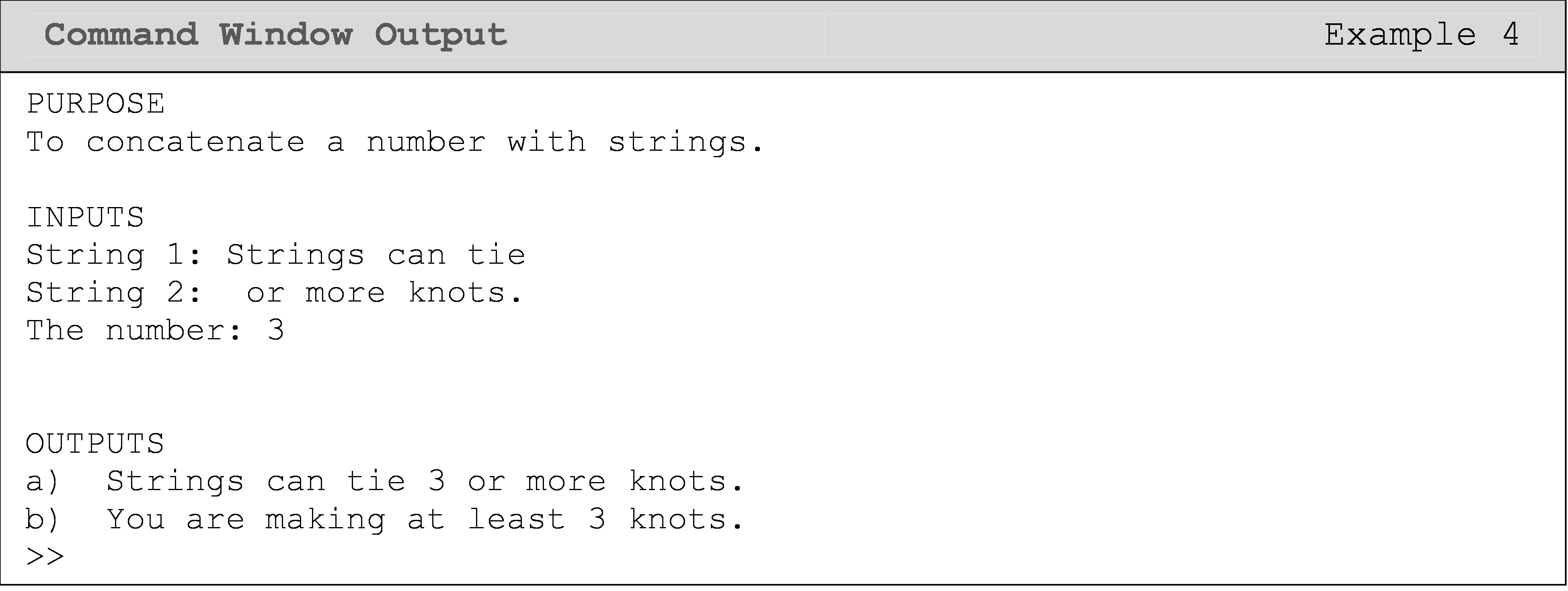## Lesson Summary of New Syntax and Programming Tools

 Task Syntax Example Usage Check the data type (class) of any variable class() class(a) Convert a numeric value to a double precision number double() double(a) Check if a variable is a numeric data type or not isnumeric() isnumeric(a) Convert a variable to a char data type (equivalent to a string) char() char(a) Check if a variable is a char data type or not ischar() ischar(a) Convert a number to a string num2str() num2str(a) Convert a string to a number str2num() str2num(a)

## Multiple Choice Quiz

(1). The num2str() function

(a)  converts a number to string

(b)  converts a string to a number

(c)  concatenates numbers and strings

(d)  concatenates strings

(2). The function to check the data type of a variable in MATLAB is

(a)  type()

(b)  class()

(c)  datatype()

(d)  var()

(3). To convert a scalar variable to a string (character data type), one should use the function

(a)  char()

(b)  scal2str()

(c)  var2str()

(d)  str2num()

(4). What data type do the conversion characters %g, %f, and %e correspond to in the fprintf() function?

(a)  char

(b)  numeric (includes double)

(c)  logical

(d)  struct

(5). To check if the stored value of a variable is numeric or not, one should use the function

(a)  ischar()

(b)  isnumeric()

(c)  isspace()

(d) isletter()

## Problem Set

(1). Define the variables in MATLAB given below and check the data type (class) of each one. Display the value of each variable along with its data type using fprintf().

myString = 'Test string'

myNum = 100

(2). Using the variable age = 30, form a string that says, “My age is 30.” Store this string in a variable called myAge.

(3). Using the functions ischar() and isnumeric(), check the variables given below to see whether or not they are the character or numeric data type. You should do both checks for each variable.

units = 'Newtons'

myNum = 50

(4). Start by checking the data type of the variable var = 83. Next, convert var to the char data type, store this string in a variable called strVar, and check the data type of this variable. Display both variables and their corresponding data types using fprintf().

(5). Using the variable age = '30', convert it to a number and store it in a variable named ageConv. Use fprintf() to print both variables with proper formatting.